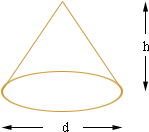Quandaries and Queries Hello,   Is there a formula to determine the cubic feet of something in a pile.  IE- I need to determine the cubic feet of a pile of mulch.  The pile comes to a peak, so the length and width decrease as the pile increases.  Thanks for your help.   Sam Hi Sam, It really depends on the shape of the pile but I think I can make a guess and an estimate. My guess is that the pile is roughly "cone shaped". That is it looks likewhere the base is approximately circular. If so then measure or estimate the height of the pile h and the diameter of the base d. The radius of the base is then r = d/2 . Th volume is then V = 1/3r2 h whereis approximately 3.1416. Penny Go to Math Central